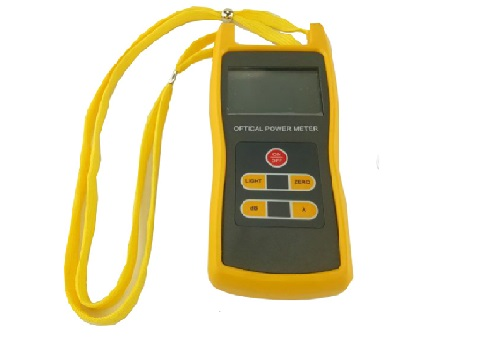﻿ What Are the Principles of the Optical Power Meter? - Hangzhou Tuolima Network Technologies Co., Ltd.

Language

+86-0571-28339001
tuolima@tuolima.com
Language
News & Blog

Products

# What Are the Principles of the Optical Power Meter?

The optical power meter is used to measure absolute optical power or relative loss of optical power through a length of fiber. In fiber optic systems, measuring optical power is fundamental, much like a multimeter in electronics.In fiber optic measurements, optical power meters are commonly used as heavy-duty meters. By measuring the absolute power of the transmitter or optical network, an optical power meter can evaluate the performance of the optical device.

Using an optical power meter in combination with a stabilized light source can measure connection loss, verify continuity, and help evaluate fiber link transmission quality.

## 1. How to choose a suitable optical power meter?

1) Select the optimal probe type and interface type

2) Evaluate calibration accuracy and manufacture calibration procedures to match your range of fiber and splice requirements.

3) Make sure the model of the optical power meter is consistent with your measurement range and display resolution.

4) It has the dB function of direct insertion loss measurement.

## 2. The working principle of the optical power meter

The unit of optical power is dbm, and its emitting and receiving optical power are listed in the manual of the optical fiber transceiver or switch.

Usually, the light emission is less than 0dbm, the minimum optical power that the receiver can receive is called sensitivity, and the unit of the maximum optical power that the optical power meter can receive minus the sensitivity value is db (dbm-dbm=db), which is called dynamic range, luminous power Subtracting the receive sensitivity is the allowable fiber attenuation value.

The value of the actual luminous power minus the actual received optical power during the test is the fiber attenuation (db).

The optimal value of the optical power received by the receiver is the maximum optical power that can be received - (dynamic range/2), but generally it is not so good.

Since the dynamic range of each optical transceiver and the optical module is different, the specific allowable attenuation of the optical fiber depends on the actual situation. Generally speaking, the allowable attenuation is about 15-30db.

Some manuals will only have two parameters, luminous power and transmission distance, and sometimes they will explain the transmission distance calculated by the fiber attenuation per kilometre, which is mostly 0.5db/km. Divide the minimum transmission distance by 0.5, which is the maximum optical power that can be received. If the received optical power is higher than this value, the optical transceiver may be burned out.

There are two ways to connect optical fibers, one is a fixed connection and the other is an active connection. The fixed connection is fusion splicing, which uses special equipment to melt the optical fiber to connect the two optical fibers together.

The optical power meter active connection is through a connector, usually a pigtail on the ODF. The advantage is that the operation is simple and the flexibility is good, and the disadvantage is that the attenuation is large. Generally speaking, the loss of an active connection is equivalent to one kilometre of fiber.

## 3. The loss of the fiber can be estimated as follows:

Including fixed and active connections, the fiber loss per kilometre is 0.5db. If there are quite a few active connections, this value can be 0.4db, but pure fiber does not include active connections and can be reduced to 0.3db. The theoretical value of pure fiber is 0.2db/km; In most cases, 0.5 is better for fiber optic power meters.

Fiber test TX and RX must be tested separately. In the case of a single fiber, only one fiber is used, so of course, only one test is required.